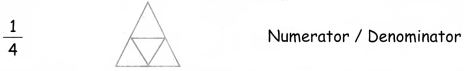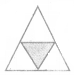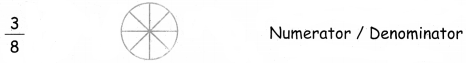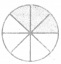Tamilnadu State Board New Syllabus Samacheer Kalvi 4th Maths Guide Pdf Term 2 Chapter 6 Fraction Ex 6.3 Textbook Questions and Answers, Notes.

## Tamilnadu Samacheer Kalvi 4th Maths Solutions Term 2 Chapter 6 Fraction Ex 6.3

I. Write the numerator and denominator for the following Numbers.
Question 1.
$$\frac { 3 }{ 7 }$$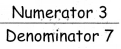Question 2.
$$\frac { 4 }{ 6 }$$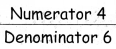Question 3.
$$\frac { 5 }{ 10 }$$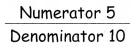Question 4.
$$\frac { 1 }{ 3 }$$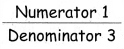II. Shade the parts according to the number and write the numerator and denominator.

Question 1.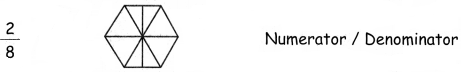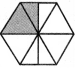Numerator = 2
Denominator = 8Question 2.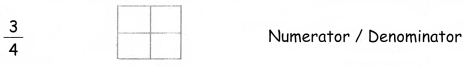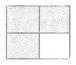Numerator = 3
Denominator = 4

Question 3.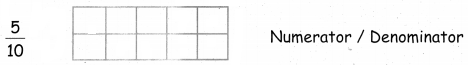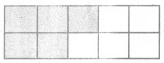Numerator = 5
Denominator = 10Question 4.